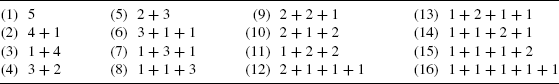Chapter 6

Compositions and Palindromes

In this chapter, we shall study different ways to write a positive integer as the sum of positive summands, or parts. For instance, for the positive integer 7, the sums 6 + 1, 3 + 1 + 3, 7, 1 + 6, 2 + 1 + 1 + 2 + 1, and 4 + 1 + 2 are six such examples, each of which is called a composition of 7. First of all, we note that here we consider 6 + 1 and 1 + 6 as different compositions of 7. So order is relevant when dealing with the compositions of a positive integer. Also, we see that the composition 3 + 1 + 3 reads the same going from left to right as it does if we read it from right to left. When this happens, we have a special type of composition that is called a palindrome.

Example 6.1: There are 16 ways to write 5 as a sum of positive integers, where the order of the summands is relevant. These representations are called the compositions of 5 and are listed in Table 6.1. [If the order is not relevant, then compositions 4 + 1 and 1 + 4 are considered to be the same partition of 5. Likewise, the three compositions 3 + 1 + 1, 1 + 3 + 1, and 1 + 1 + 3 correspond to only one partition of 5. Overall, the 16 compositions of 5 determine seven partitions of 5.]

Table 6.1In order to obtain a formula for the number of compositions of an arbitrary positive integer n, let us look at the compositions of 5 once again. In particular, consider the following ...

Get Fibonacci and Catalan Numbers: An Introduction now with the O’Reilly learning platform.

O’Reilly members experience live online training, plus books, videos, and digital content from nearly 200 publishers.